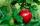# Expression of a variable from formula - examples - page 2

1. Square diagonalCalculate length of the square diagonal if the perimeter is 476 cm.
2. IS trapezoidCalculate the length of diagonal u and height v of isosceles trapezoid ABCD, whose bases have lengths a = |AB| = 37 cm, c = |CD| = 29 cm and legs b = d = |BC| = |AD| = 28 cm.
3. TheaterThe theater has in each row with 19 seats. Ticket to the first 10 rows is for 30 USD. In next rows is for 15 USD. The theater was completely sold out. Revenue was 12255 USD. How many rows are in the theater?
4. ConeCircular cone of height 15 cm and volume 5699 cm3 is at one-third of the height (measured from the bottom) cut by a plane parallel to the base. Calculate the radius and circumference of the circular cut.
5. Sea waterMixing 34 kg of sea water with 34 kg rainwater is created water containing 3.4% salt. How many percent sea water contains salt?
6. Floating barrelBarrel (cylinder shape) floats on water, top of barrel is 8 dm above water and the width of surfaced barrel part is 23 dm. Barrel length is 24 dm. Calculate the volume of the barrel.
7. R TrapeziumRectangular trapezium has bases 12 and 5 and area 84 cm2. What is its perimeter?
8. Arc and segmentCalculate the length of circular arc l, area of the circular arc S1 and area of circular segment S2. Radius of the circle is 33 and corresponding angle is ?.
9. Prism XThe prism with the edges of the lengths x cm, 2x cm and 3x cm has volume 20250 cm3. What is the area of surface of the prism?
10. ISO triangleCalculate the area of an isosceles triangle KLM if the length of its sides are in the ratio k:l:m = 4:4:3 and has perimeter 377 mm.
11. GardenFather dig up the garden in 9 hours. Son in 13 hours. How many hours take dig up the garden together?
12. Rhombus 2Calculate the area of rhombus which has a height v=48 mm and shorter diagonal u = 60 mm long.
13. CircleHow big is area of circle if its circumference is 80.6 cm?
14. Right isoscelesCalculate area of the isosceles right triangle which perimeter is 41 cm.
15. TrianglesEquilateral triangle with side 40 cm has the same perimeter as an isosceles triangle with arm of 45 cm. Calculate the base x of an isosceles triangle.
16. Square diagonalCalculate the length of diagonal of the square with side a = 23 cm.
17. GardenTrapezoid garden has parallel sides 19 m and 24 m. Its area is 193.5 square meters. What is the width of the garden?
18. Cylinder - hCylinder volume is 215 cm3. Base radius is 2 cm. Calculate the height of the cylinder.
19. Truncated coneCalculate the height of the rotating truncated cone with volume V = 1115 cm3 and a base radii r1 = 7.9 cm and r2 = 9.7 cm.
20. Right triangleCalculate the missing side b and interior angles, perimeter and area of ​​a right triangle if a=10 cm and hypotenuse c = 16 cm.

Do you have an interesting mathematical example that you can't solve it? Enter it, and we can try to solve it.

To this e-mail address, we will reply solution; solved examples are also published here. Please enter e-mail correctly and check whether you don't have a full mailbox.